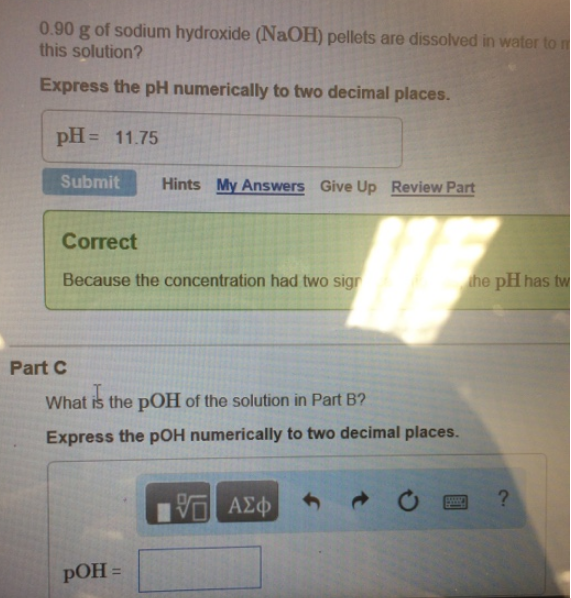Chemistry Practice Problems pH and pOH Practice Problems Solution: 0.90 g of sodium hydroxide (NaOH) pellets are diss...

🤓 Based on our data, we think this question is relevant for Professor Hurst's class at GS.

# Solution: 0.90 g of sodium hydroxide (NaOH) pellets are dissolved in water to this solution? Express the pH numerically to two decimal places. pH = 11.75 What is the pOH of the solution in Part B? Express the pOH numerically to two decimal places.

###### Problem

0.90 g of sodium hydroxide (NaOH) pellets are dissolved in water to this solution? Express the pH numerically to two decimal places. pH = 11.75 What is the pOH of the solution in Part B? Express the pOH numerically to two decimal places.View Complete Written Solution

pH and pOH

pH and pOH

#### Q. At 25 C, how many dissociated OH ions are there in 1219 mL of an aqueous solution whose pH is 1.56?

Solved • Mon May 21 2018 07:28:53 GMT-0400 (EDT)

pH and pOH

#### Q.  Calculate the poH of a 0.0321 M HCIO4 solution at the following temperatures

Solved • Fri May 04 2018 18:34:41 GMT-0400 (EDT)

pH and pOH

#### Q. 1. Three solutions have the following pH:      pH = 4.3      pH = 6.8      pH = 11.5      a) Which solution contains the highest H3O+ ion concentratio...

Solved • Fri May 04 2018 13:14:02 GMT-0400 (EDT)

pH and pOH

#### Q. What are the concentrations of hydrogen ion and hydroxide ion in household ammonia, an aqueous solution of NH3 that has a pH of 12.3?

Solved • Tue May 01 2018 17:23:50 GMT-0400 (EDT)